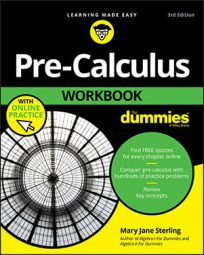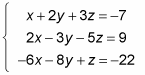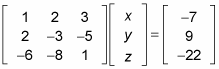##### Pre-Calculus Workbook For DummiesIn a system of linear equations, where each equation is in the form Ax + By + Cz + . . . = K, you can represent the coefficients of this system in matrix, called the coefficient matrix.

If all of the variables line up with one another vertically, then the first column of the coefficient matrix is dedicated to all the coefficients of the first variable, the second row is for the second variable, and so on. Each row then represents the coefficients of each variable in the order that they appear in the system of equations.

Through a couple of different processes, you can manipulate the coefficient matrix in order to make the solutions easier to find.

Solving a system of equations using a matrix is a great method, especially for larger systems (with more variables and more equations). However, these methods work for systems of all sizes, so you have to choose which method is appropriate for which problem.

You can write any system of equations as a matrix. Take a look at the following system:To express this system in matrix form, you follow three simple steps:

1. Write all the coefficients in one matrix first.

This is called a coefficient matrix.

2. Multiply this matrix with the variables of the system set up in another matrix.

This is sometimes called the variable matrix.

This is sometimes called the answer matrix.

The setup appears as follows:Notice that the coefficients in the matrix go in order — you see a column for x, y, and z.